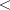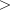JMSLTM Numerical Library 7.2.0
com.imsl.chart

## Class DrawMap

• ```public class DrawMap
extends Draw```
Creates an HTML client-side imagemap from a chart tree. Entries in the imagemap correspond to nodes that define the HREF attribute.
Chart Programmer's Guide: Servlets

• ### Fields inherited from class com.imsl.chart.Draw

`currentType, ERROR_BAR, FILL, fillColor, fillOutlineColor, fillOutlineType, fillPaint, fillType, graphics, haveErrorBarProperties, haveFillProperties, haveImageProperties, haveLineProperties, haveMarkerProperties, haveTextProperties, IMAGE, imageObserver, LAST, LINE, lineColor, lineDashPattern, lineWidth, MARKER, MARKER_SCALE, markerColor, markerDashPattern, markerSize, markerThickness, markerType, node, NONE, outline, path, RADIAN, scaleFont, TEXT, textAngle, textColor, textFont`
• ### Constructor Summary

Constructors
Constructor and Description
```DrawMap(Graphics graphics, Dimension bounds)```
Contructs a DrawMap object.
• ### Method Summary

Methods
Modifier and Type Method and Description
`protected void` ```circle(int x, int y, int r)```
Sets a circle as the target.
`void` ```drawArc(int x, int y, int width, int height, int startAngle, int arcAngle)```
Draws the outline of a circular or elliptical arc covering the specified rectangle.
`void` ```drawErrorBar(int x0, int y0, int x1, int y1, int flag)```
Draw an error bar.
`void` ```drawImage(Image image, int x, int y)```
Draw Image
`void` ```drawLine(int x0, int y0, int x1, int y1)```
Draw a line from (x0,y0) to (x1,y1).
`void` ```drawMarker(int x, int y)```
Draw a marker.
`protected Dimension` ```drawText(Text text, int x, int y, boolean dimensionOnly)```
Draws a text object.
`void` `endErrorBar()`
Stop drawing an error bar.
`void` `endFill()`
Stop drawing a filled region.
`void` `endImage()`
Stop drawing an image.
`void` `endLine()`
Finish drawing lines.
`void` `endMarker()`
Finish drawing markers.
`void` `endText()`
Stop drawing text.
`void` ```fillArc(int x, int y, int width, int height, int startAngle, int arcAngle)```
Fills a circular or elliptical arc covering the specified rectangle.
`void` ```fillPolygon(int[] xpoints, int[] ypoints, int npoints)```
Fill a polygon.
`void` `fillPolygon(Polygon polygon)`
Fill a polygon defined by a `Polygon` object.
`void` ```fillRectangle(int x, int y, int width, int height)```
Fill a rectangle.
`protected String` `getALT()`
Returns the current ALT string.
`protected String` `getHREF()`
Returns the current HREF string.
`String` `getMap()`
Returns the body of the HTML imagemap.
`int` `getTolerance()`
Get the minimum distance that an event can be from a point or a line and still be considered a hit.
`protected void` ```poly(int[] x, int[] y)```
Sets a polygon as the target.
`protected void` ```rect(int x, int y, int w, int h)```
Sets a rectangle as the target.
`void` `setNode(ChartNode node)`
Set the current ChartNode.
`void` `setTolerance(int tolerance)`
Set the minimum distance that an event can be from a point or a line and still be considered a hit.
`void` `startErrorBar()`
Start drawing an error bar.
`void` `startFill()`
Start drawing a filled region.
`void` `startImage()`
Start drawing an image.
`void` `startLine()`
Start drawing lines.
`void` `startMarker()`
Start drawing markers.
`void` `startText()`
Start drawing text.
`void` ```translate(int x, int y)```
Translates the origin to the point (x,y)
• ### Methods inherited from class com.imsl.chart.Draw

`check, drawClippedImage, drawRotatedText, drawText, drawText, getClipBounds, getDeviceMarkerSize, getScaleFont, getSize, setClip, setScaleFont, start, stop`
• ### Methods inherited from class java.lang.Object

`clone, equals, finalize, getClass, hashCode, notify, notifyAll, toString, wait, wait, wait`
• ### Constructor Detail

• #### DrawMap

```public DrawMap(Graphics graphics,
Dimension bounds)```
Contructs a DrawMap object.
Parameters:
`graphics` - is the graphics context in which to draw.
`bounds` - is the size of the chart to be drawn.
• ### Method Detail

• #### circle

```protected void circle(int x,
int y,
int r)```
Sets a circle as the target.
Parameters:
`x` - is the x-coordinate of the center of the circle
`y` - is the y-coordinate of the center of the circle
`r` - is the radius of the circle
• #### drawArc

```public void drawArc(int x,
int y,
int width,
int height,
int startAngle,
int arcAngle)```
Draws the outline of a circular or elliptical arc covering the specified rectangle. The center of the arc is center of this rectangle.
Overrides:
`drawArc` in class `Draw`
Parameters:
`x` - An `int` which specifies the x of the rectangle.
`y` - An `int` which specifies the y of the rectangle origin.
`width` - An `int` which specifies the width of the rectangle.
`height` - An `int` which specifies the height of the rectangle.
`startAngle` - An `int` which specifies the start angle in degrees. startAngle = 0 is equivalent to the 3-o'clock position.
`arcAngle` - An `int` which specifies the arcAngle. drawArc draws the arc from startAngle to startAngle+arcAngle. A positive arcAngle indicates a counter-clockwise rotation. A negative arcAngle implies a clockwise rotation.
• #### drawErrorBar

```public void drawErrorBar(int x0,
int y0,
int x1,
int y1,
int flag)```
Draw an error bar.
Overrides:
`drawErrorBar` in class `Draw`
Parameters:
`x0` - an `int` which specifies the x-coordinate of the beginning reference point
`y0` - an `int` which specifies the y-coordinate of the beginning reference point
`x1` - an `int` which specifies the x-coordinate of the ending reference point
`y1` - an `int` which specifies the y-coordinate of the ending reference point
`flag` - an `int` that indicates which caps to draw (0=none, 1=bottom, 2=top, 3=both).
• #### drawImage

```public void drawImage(Image image,
int x,
int y)```
Draw Image
Overrides:
`drawImage` in class `Draw`
Parameters:
`image` - the `Image` object to be drawn
`x` - an `int` which specifies the x-coordinate of the reference point
`y` - an `int` which specifies the y-coordinate of the reference point
• #### drawLine

```public void drawLine(int x0,
int y0,
int x1,
int y1)```
Draw a line from (x0,y0) to (x1,y1).
Overrides:
`drawLine` in class `Draw`
Parameters:
`x0` - an `int` which specifies the x0 of the line origin, (x0,y0)
`y0` - an `int` which specifies the y0 of the line origin, (x0,y0)
`x1` - an `int` which specifies the x1 of the line destination, (x1,y1)
`y1` - an `int` which specifies the y1 of the line destination, (x1,y1)
• #### drawMarker

```public void drawMarker(int x,
int y)```
Draw a marker.
Overrides:
`drawMarker` in class `Draw`
Parameters:
`x` - an `int` which specifies the x of the marker destination, (x,y)
`y` - an `int` which specifies the y of the marker destination, (x,y)
• #### drawText

```protected Dimension drawText(Text text,
int x,
int y,
boolean dimensionOnly)```
Description copied from class: `Draw`
Draws a text object. The angle of the string is given by textAngle. Consider the horizontally and vertically aligned bounding box around the string. The box below corresponds to textAngle == 45.

```        *--*--*
|    o|
|   l |
*  l  *
| e   |
|H    |
*--*--*
```

The reference point corresponds to one of the 8 starred points on the bounding box, as indicated by the "alignment" attribute" in the text object.

Overrides:
`drawText` in class `Draw`
Parameters:
`text` - a `Text` object to be drawn.
`x` - an `int` which specifies the x-coordinate of the reference point.
`y` - an `int` which specifies the y-coordinate of the reference point.
`dimensionOnly` - a `boolean` which is true if only the bounding box is to be computed and no text actually drawn.
Returns:
the dimension of the bounding box.
• #### endErrorBar

`public void endErrorBar()`
Description copied from class: `Draw`
Stop drawing an error bar.
Overrides:
`endErrorBar` in class `Draw`
• #### endFill

`public void endFill()`
Description copied from class: `Draw`
Stop drawing a filled region.
Overrides:
`endFill` in class `Draw`
• #### endImage

`public void endImage()`
Description copied from class: `Draw`
Stop drawing an image.
Overrides:
`endImage` in class `Draw`
• #### endLine

`public void endLine()`
Description copied from class: `Draw`
Finish drawing lines.
Overrides:
`endLine` in class `Draw`
• #### endMarker

`public void endMarker()`
Description copied from class: `Draw`
Finish drawing markers.
Overrides:
`endMarker` in class `Draw`
• #### endText

`public void endText()`
Description copied from class: `Draw`
Stop drawing text.
Overrides:
`endText` in class `Draw`
• #### fillArc

```public void fillArc(int x,
int y,
int width,
int height,
int startAngle,
int arcAngle)```
Fills a circular or elliptical arc covering the specified rectangle. The center of the arc is center of this rectangle.
Overrides:
`fillArc` in class `Draw`
Parameters:
`x` - An `int` which specifies the x of the rectangle.
`y` - An `int` which specifies the y of the rectangle origin.
`width` - An `int` which specifies the width of the rectangle.
`height` - An `int` which specifies the height of the rectangle.
`startAngle` - An `int` which specifies the start angle in degrees. `startAngle = 0` is equivalent to the 3-o'clock position.
`arcAngle` - An `int` which specifies the `arcAngle`. `drawArc` draws the arc from `startAngle` to `startAngle+arcAngle`. A positive `arcAngle` indicates a counter-clockwise rotation. A negative `arcAngle` implies a clockwise rotation.
• #### fillPolygon

```public void fillPolygon(int[] xpoints,
int[] ypoints,
int npoints)```
Fill a polygon.
Overrides:
`fillPolygon` in class `Draw`
Parameters:
`xpoints` - an `int` array which contains the abscissae of the points which define the polygon
`ypoints` - an `int` array which contains the ordinates of the points which define the polygon
`npoints` - an `int` which specifies the number of points
• #### fillPolygon

`public void fillPolygon(Polygon polygon)`
Fill a polygon defined by a `Polygon` object.
Overrides:
`fillPolygon` in class `Draw`
Parameters:
`polygon` - a `Polygon` object which specifies the polygon to be filled
• #### fillRectangle

```public void fillRectangle(int x,
int y,
int width,
int height)```
Fill a rectangle.
Overrides:
`fillRectangle` in class `Draw`
Parameters:
`x` - an `int` which specifies the abscissa of the origin of the rectangle
`y` - an `int` which specifies the ordinate of the origin of the rectangle
`width` - an `int` which specifies the width of the rectangle
`height` - an `int` which specifies the height of the rectangle
• #### getALT

`protected String getALT()`
Returns the current ALT string.
• #### getHREF

`protected String getHREF()`
Returns the current HREF string.
• #### getMap

`public String getMap()`
Returns the body of the HTML imagemap.
Returns:
the body of the HTML client-side imagemap. The actualmapand/maptags are not included, so that the client code can more easily add attributes to themaptag.
• #### getTolerance

`public int getTolerance()`
Get the minimum distance that an event can be from a point or a line and still be considered a hit.
Returns:
an `int` which specifies the minimum distance that an event can be from a point or a line and still be considered a hit
• #### poly

```protected void poly(int[] x,
int[] y)```
Sets a polygon as the target.
Parameters:
`x` - is an array containing the x-coordinates of the polygon.
`y` - is an array containing the y-coordinates of the polygon.
• #### rect

```protected void rect(int x,
int y,
int w,
int h)```
Sets a rectangle as the target.
Parameters:
`x` - is the x-coordinate of the left edge of the rectangle
`y` - is the y-coordinate of the top edge of the rectangle
`w` - is the width of the rectangle
`h` - is the height of the rectangle
• #### setNode

`public void setNode(ChartNode node)`
Set the current ChartNode. This is used to get drawing attributes from the tree.
Overrides:
`setNode` in class `Draw`
Parameters:
`node` - a `ChartNode` object
• #### setTolerance

`public void setTolerance(int tolerance)`
Set the minimum distance that an event can be from a point or a line and still be considered a hit.
Parameters:
`tolerance` - an `int` which specifies the minimum distance that an event can be from a point or a line and still be considered a hit
• #### startErrorBar

`public void startErrorBar()`
Description copied from class: `Draw`
Start drawing an error bar.
Overrides:
`startErrorBar` in class `Draw`
• #### startFill

`public void startFill()`
Description copied from class: `Draw`
Start drawing a filled region.
Overrides:
`startFill` in class `Draw`
• #### startImage

`public void startImage()`
Description copied from class: `Draw`
Start drawing an image.
Overrides:
`startImage` in class `Draw`
• #### startLine

`public void startLine()`
Start drawing lines.
Overrides:
`startLine` in class `Draw`
• #### startMarker

`public void startMarker()`
Start drawing markers.
Overrides:
`startMarker` in class `Draw`
• #### startText

`public void startText()`
Description copied from class: `Draw`
Start drawing text.
Overrides:
`startText` in class `Draw`
• #### translate

```public void translate(int x,
int y)```
Translates the origin to the point (x,y)
Overrides:
`translate` in class `Draw`
Parameters:
`x` - an `int` which specifies the x of the new origin
`y` - an `int` which specifies the y of the new origin
JMSLTM Numerical Library 7.2.0